# Summary functions

## Vector functions

The package adds the following vector functions

# sample_mode returns the statistical mode
sample_mode(c(1, 2, 2))
sample_mode(c(1, 2))
sample_mode(c(NA, NA, 1))
sample_mode(c(NA, NA, 1), na.rm = TRUE)

# bin creates integer variable for quantile categories (corresponds to Stata xtile)
v <- c(NA, 1:10)
bin(v, n_quantiles = 3) # 3 groups based on terciles
bin(v, probs = c(0.3, 0.7)) # 3 groups based on two quantiles
bin(v, cutpoints = c(2, 3)) # 3 groups based on two cutpoints

# winsorize (default based on 5 x interquartile range)
v <- c(1:4, 99)
winsorize(v)
winsorize(v, replace = NA)
winsorize(v, probs = c(0.01, 0.99))
winsorize(v, cutpoints = c(1, 50))

# demean on multiple groups (ie multiple fixed effects)
demean(c(1,2), fe = c(1,1))
demean(c(NA,2), fe = list(c(1,2), c(1,3)))
demean(c(1,2), fe = list(c(NA,2), c(1,3)))

## data.table functions

### Keep and drop

# setkeep keeps certain columns inplace
DT <- data.table(
id = c(1,2),
v1 = c(1,1),
v2 = c(2,1)
)
setkeep(DT, list(id, v2))
setkeep(DT, -id)
setdrop(DT, v1)

### Summarize

# sum_up prints detailed summary statistics (corresponds to Stata summarize)
N <- 100
DT <- data.table(
id = 1:N,
v1 = sample(5, N, TRUE),
v2 = sample(1e6, N, TRUE)
)
sum_up(DT)
sum_up(DT, v2, d = TRUE)
sum_up(DT, starts_with("v"), by = v1)

# tab returns cross tabulation table (faster than tabulate)
tab(DT, id)
tab(DT, id, v1, w = v2)

# duplicates returns duplicated rows
DT <- data.table(a = rep(1:2, each = 3), b = 1:6)
duplicates(DT, by = a)
duplicates(DT, by = list(a,b))

## Visual exploration

graph is a wrapper for ggplot2 functionalities, useful for interactive exploration of datasets

N <- 10000
DT <- data.table(
id = sample(c("id1","id2","id3"), N, TRUE),
v1 = sample(c(1:5), N, TRUE),
v2 = rnorm(N, sd = 20),
v3 = sample(runif(100, max=100), N, TRUE)
)
DT[, v4 := (id=="id1")* v2 + rnorm(N, sd = 5)]
graph(DT)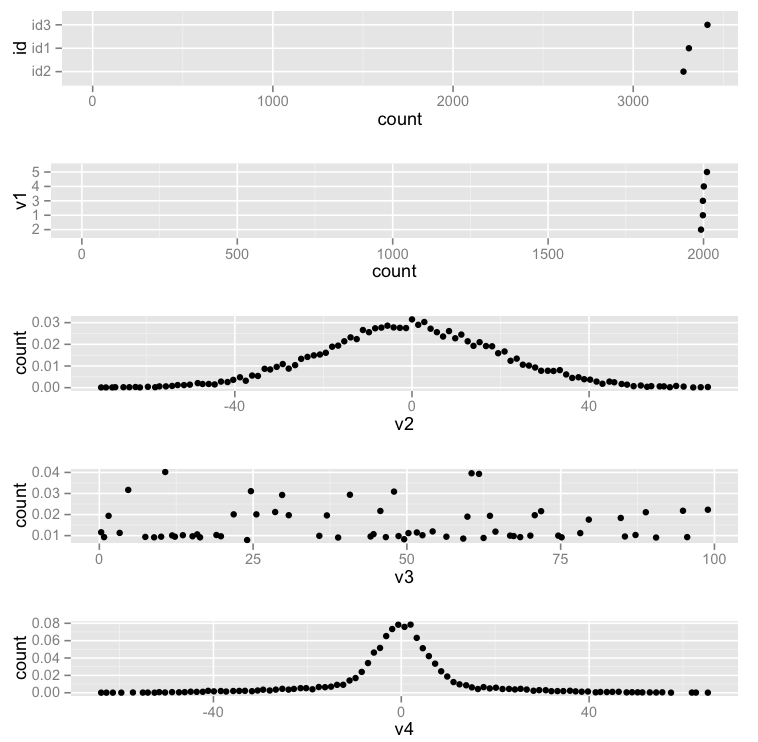graph(DT, by = id)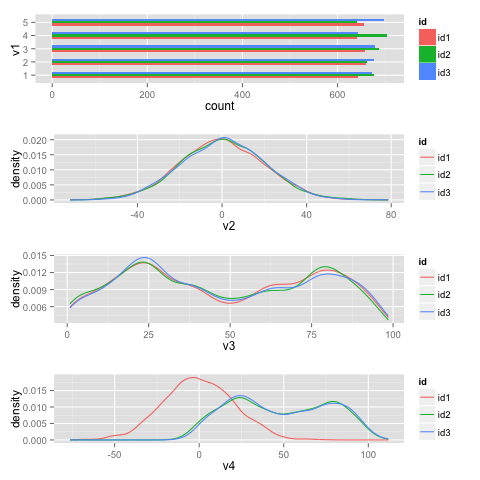graph(DT, by = id, type = "boxplot")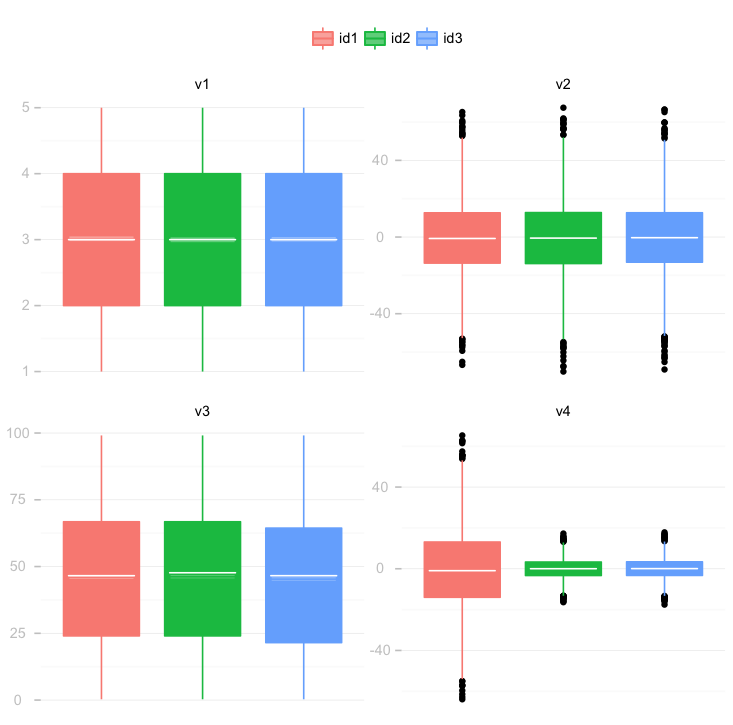graph(DT, list(v3, v4), along_with = v2)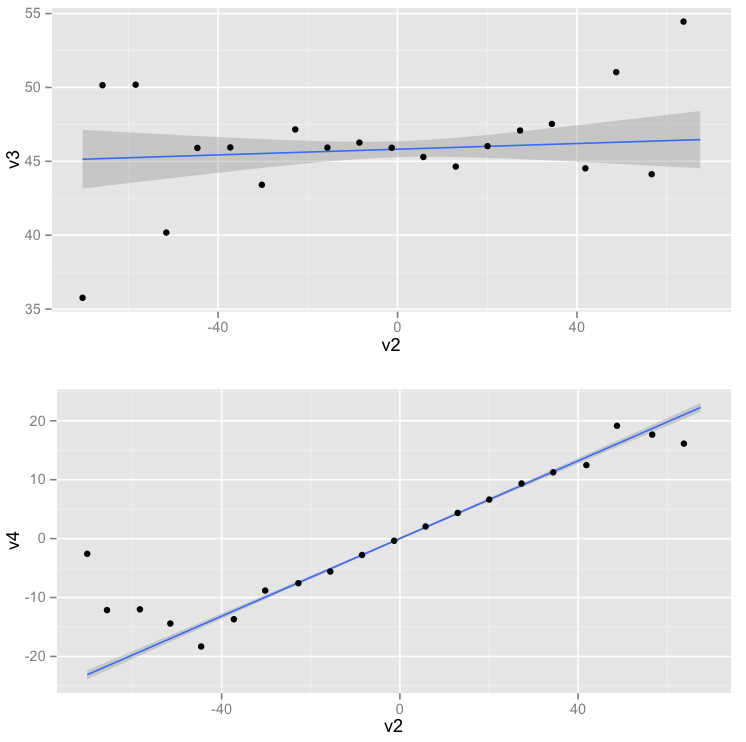graph(DT, list(v3, v4), along_with = v2, by = id, type = "loess")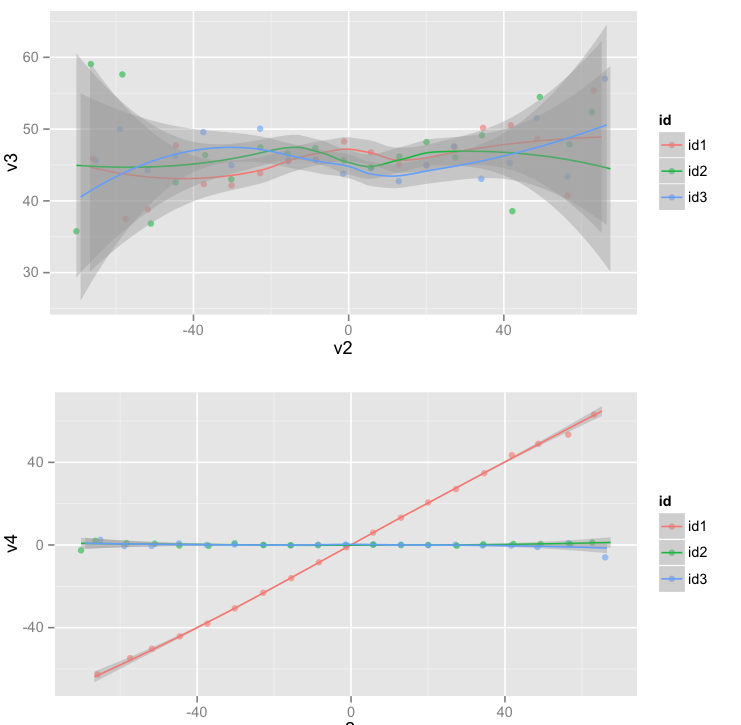## Join

join is a wrapper for data.table merge functionalities.

• The option “kind” specifies the kind of join based on SQL syntax. Possible kinds are : left, right, inner, outer, semi, anti and cross.
Stata statar
merge v1 join(x, y, kind = “outer”)
merge v1, keep(master matched) join(x, y, kind = “left”)
merge v1, keep(matched using) join(x, y, kind = “right”)
merge v1, keep(matched) join(x, y, kind = “inner”)
merge v1, keep(matched) keepusing(v1) join(x, y, kind = “semi”)
merge v1, keep(master) keepusing(v1) join(x, y, kind = “anti”)
crossby join(x, y, kind = “cross”)
• The option “check” checks there are no duplicates in the master or using data.tables (as in Stata).

r # merge m:1 v1 join(x, y, kind = "outer", check = m~1) - The option “gen” specifies the name of a new variable that identifies non matched and matched rows (as in Stata).

r # merge m:1 v1, gen(_merge) join(x, y, kind = "outer", gen = "_merge")

• The option “inplace” specifies whether the dataset x should be merged in place. It is only available for left joins, when y has no duplicates (for now).

## Syntax

Functions with the prefix set modify the input data.table in place. Function selects variables similarly to dplyr syntax. Each function has a version that accepts strings, formulas or quoted expressions : its name is the original function’s name with the suffix _ (see the dplyr vignette for more details). For instance, the SE version of sum_up is sum_up_.

# NSE version
sum_up(DT, list(v2, v3), by = list(id,v1))
# SE version
sum_up_(DT, c("v2","v3"), by = c("id","v1"))